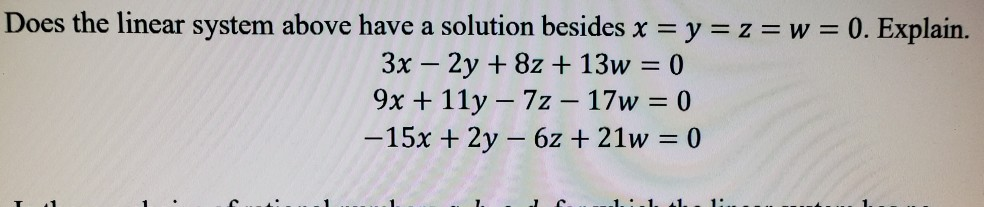Home / Answered Questions / Other / does-the-linear-system-above-have-a-solution-besides-0-explain-3-2-8-13-0-9-11-7-17-0-15-2-6-21-0-aw460

# (Solved): Does The Linear System Above Have A Solution Besides ð‘¥ = ð‘¦ = ð‘§ = ð‘¤ = 0. Explain. 3ð‘¥ â...

Does the linear system above have a solution besides ð‘¥ = ð‘¦ = ð‘§ = ð‘¤ = 0. Explain.

3ð‘¥ âˆ’ 2ð‘¦ + 8ð‘§ + 13ð‘¤ = 0

9ð‘¥ + 11ð‘¦ âˆ’ 7ð‘§ âˆ’ 17ð‘¤ = 0

âˆ’15ð‘¥ + 2ð‘¦ âˆ’ 6ð‘§ + 21ð‘¤ = 0Does the linear system above have a solution besides x = y = z = w = 0. Explain. 3x â€“ 2y + 8z + 13w = 0 9x + 11y â€“ 72 â€“ 17w = 0 -15x + 2y - 6x + 21w = 0 1. 1 1. 1

We have an Answer from Expert